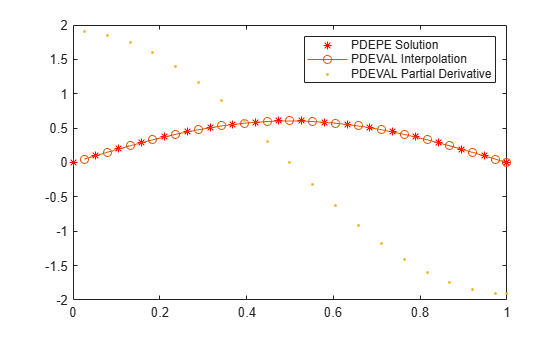Documentation

# pdeval

Interpolate numerical solution of PDE

## Syntax

``[u,dudx] = pdeval(m,xmesh,usol,xq)``

## Description

example

````[u,dudx] = pdeval(m,xmesh,usol,xq)` interpolates a numerical solution returned by `pdepe` at new query points `xq`, and returns the interpolated values of the solution `u` and their partial derivative `dudx`. The `m`, `xmesh`, and `usol` arguments are reused from a previous call to `pdepe`: The numerical solution produced by ```sol = pdepe(m,@pdefun,@pdeic,@pdebc,xmesh,tspan)``` uses the coordinate symmetry `m` and spatial mesh `xmesh` to return a 3-D matrix of the solution values `sol`. Reuse the `m` and `xmesh` inputs used to calculate the solution when you call `pdeval`.The input vector `usol = sol(i,:,k)` is the value of component `k` of the solution at time `tspan(i)`. When there is only one solution component, `usol` is a row extracted from the solution matrix `usol = sol(i,:)`. ```

## Examples

collapse all

Use `pdepe` to solve a partial differential equation, and then use `pdeval` to evaluate the solution at additional points.

Solve PDE

Use `pdepe` to solve the `pdex1` example problem. You can type `edit pdex1` to see more details on the problem, or see `pdepe` for details on the PDE solution process. The required functions to solve the `pdex1` problem are included at the end of this example as local functions.

```m = 0; x = linspace(0,1,20); t = linspace(0,2,5); sol = pdepe(m,@pdex1pde,@pdex1ic,@pdex1bc,x,t);```

Interpolate Solution

The solution `sol` generated by `pdepe` uses 20 points for `x`, evenly spaced between 0 and 1. Create a vector of query points that are located midway between the points used by `pdepe`.

```xq = x; xq(1:end-1) = xq(1:end-1) + diff(xq)./2;```

Use `pdeval` to interpolate the solution at the query points. Since there is only one solution component, you can extract a row from `sol` to operate on, such as `sol(2,:)`.

`[u,dudx] = pdeval(m,x,sol(2,:),xq);`

Plot the solution computed by `pdepe`, as well as the interpolated solution and its partial derivative computed by `pdeval`.

```plot(x,sol(2,:),'r*') hold on plot(xq,u,'-o') plot(xq,dudx,'.') hold off legend('PDEPE Solution', 'PDEVAL Interpolation', 'PDEVAL Partial Derivative')```Local Functions

Listed here are the local helper functions that the PDE solver `pdepe` calls to calculate the solution.

```function [c,f,s] = pdex1pde(x,t,u,DuDx) c = pi^2; f = DuDx; s = 0; end % ------------------------------------------------------ function u0 = pdex1ic(x) u0 = sin(pi*x); end % ------------------------------------------------------ function [pl,ql,pr,qr] = pdex1bc(xl,ul,xr,ur,t) pl = ul; ql = 0; pr = pi * exp(-t); qr = 1; end```

## Input Arguments

collapse all

Coordinate symmetry used with `pdepe`, specified as one of the values in this table. Specify the same coordinate symmetry you used in the initial call to `pdepe`.

ValueSymmetry

`0`

Slab/Cartesian

`1`

Cylindrical

`2`

Spherical

Spatial mesh used with `pdepe`, specified as a vector `[x0 x1 ... xn]` containing the points at which a numerical solution was computed. Specify the same spatial mesh you used in the initial call to `pdepe`.

Data Types: `single` | `double`

Extracted solution component, specified as a vector of values computed by `pdepe` for one solution component at a particular time.

`pdepe` returns the solution in a 3-D array `sol`, where `sol(i,j,k)` approximates the `k`th component of the solution ${\mathit{u}}_{\mathit{k}}$ evaluated at time `t(i)` and spatial point `xmesh(j)`. You can create the input `usol` with the command `usol = sol(i,:,k)`, where `sol(i,:,k)` is the value of component `k` of the solution at time `tspan(i)`, evaluated on the entire spatial mesh `xmesh`. When there is only one solution component, `usol` is a row extracted from the solution matrix ```usol = sol(i,:)```.

Example: `usol = sol(10,:,2)` extracts the second solution component calculated at time `tspan(10)`.

Example: `usol = sol(5,:)` extracts the solution calculated at time `tspan(5)`.

Data Types: `single` | `double`
Complex Number Support: Yes

Query points, specified as a scalar or vector of x-coordinates. The points specified in `xq` must lie within the interval `[min(xmesh) max(xmesh)]`.

Typically, the points in `xq` are specified between the values in `xmesh` to enable `pdeval` to evaluate the solution produced by `pdepe` on a finer mesh.

Example: `xq = linspace(0,1,100)` specifies 100 evenly spaced query points between 0 and 1.

Data Types: `single` | `double`
Complex Number Support: Yes

## Output Arguments

collapse all

Interpolated solution, returned as a vector with the same size as `xq`.

Partial derivative of interpolated solution, returned as a vector with the same size as `xq`.

## Tips

• `pdeval` evaluates the partial derivative $\frac{\partial u}{\partial x}$ rather than the flux $f\left(x,t,u,\frac{\partial u}{\partial x}\right)$. Although the flux is continuous, the partial derivative can have a jump at a material interface.